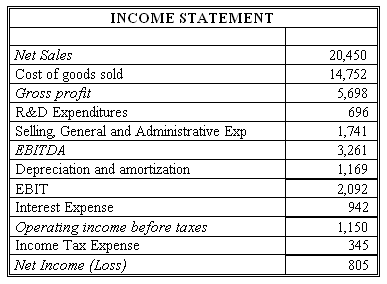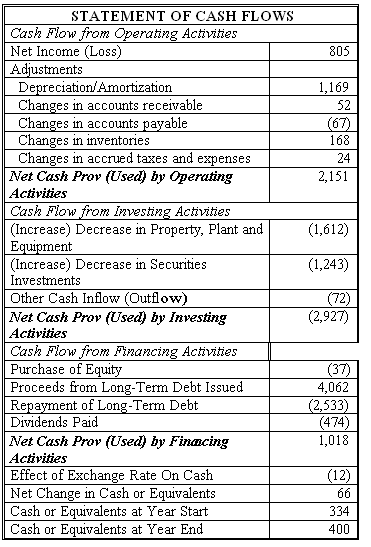### CFA Practice Question

There are 534 practice questions for this study session.

### CFA Practice Question

Connie's Sporting Goods (CSG) has net income of \$805 million for 2016. Using information from CSG's financial statements below, find what FCFF and FCFE should be for CSG. Assume the income tax rate is 30%.Correct Answer: \$1,198 million and \$2,068 million

FCFF = NI + NCC + Int (1 - Tax rate) - FCInv - WCInv
= 805 + 1169 + 942 x (1 - 0.3) - 1612 - (-52 + 67 - 168 - 24) = \$1,198 million

FCFE = NI + NCC + Net borrowing - FCInv - WCInv
= 805 + 1169 + (4062 - 2533) - 1612 - (-52 + 67 - 168 - 24) = \$2,068 million

We can also find FCFE from FCFF. FCFE = FCFF - Int (1 - Tax rate) + Net borrowing = 1198 - 942 (1 - 0.3) + (4062 - 2533) = \$2,068 million

User Comment
danlan2 Also FCFF
=CFO+Int(1-Tax rate)-FCInv
=2151+942*(1-0.3)-1612
=1198

and FCFE
=CFO+Net Borrowing-FCInv
=2151+(4062-2533)-1612
=2068
Oarona Thanks danlan2
Leese Above FCFF was given as 805+1169+942*(1-0.3)-1612-(-52+67-168-24)=1198.
Am a little confused. Shouldnt working capital be -52-67-168-24=-311 (a decrease in accounts payable means we paid out more money, right?

So FCFF=
NI 805
Depr 1169
Interest 942(1-0.3)
FCInv (1612)
WCInv (311)
FCFF 710

Am I missing something? I followed example on pp 387-389 CFAI vol 4 2010. Can anyone help?
Leese I think I have answered my own question: here we are adding back the change in accts rec so they must have decreased and we are deducting the change in accts payable so they must have decreased also. Overall working capital has decreased, so we add it back to find FCFF. Is that correct?
vatsa Leese, calculate FCFF and FCFE startin from CFO.
Your life will be lot easier and can save time as well, it will help in exam.
johntan1979 Yes, save time, save life, start with the shorter formula i.e. CFO

And by the way, I suppose equity borrowing is not included in net borrowing which is why the \$37 stock buyback is not counted?
hks101 The idea of FCFE is how much cash flow is available to equity holders. Borrowing money from equity holders is not earning money and returning to equity holders.
Stock buyback is an example of FCFE usage, just like issuing dividends.
robertucla Learn both ways. You never know what test is going to through at you on game day.
robbiecow FCFF can be derived from
FCF + Interest Expense ...captures everything, but the needed term thats used in all the calculations below (Interest)
NI + NCC + Int*(1-t) - WCinv - FCinv ...
EBIT(1-t) + NCC - WCinv - FCinv ...EBIT has 4 letters so there are 4 inputs
EBITDA(1-t) + NCC(t) - WCinv -FCinv ...same as above
CFO + Int*(1-t) - FCinv ...CFO has 3 letters so there are 3 inputs

FCFE can be derived from
FCF + Net Borrowing ... captures everything, but the needed term thats used in all the calculations below (Net Borrowing)
NI + NCC + Net Borrowing - WCinv - FCinv
NI - (Capex - Depr.) - WCinv+ (New Debt - Debt Repayment)
CFO + Net Borrowing - FCinv
Debt Ratio: NI - (1-DR)*(FVinv - Depr.) - (1-DR)*WCinv
FCFF - Int*(1-t) + Net Borrowing
fzhou Can someone explain why WC Inv = -52+67-168-24? I thought it's the other way around = 52-67+168+24. According to the reading, WC Inv equals to the increase in short-term operating assets net of operating liabilities, right?
linzlinked **fzhou, you are right, but the WC Inv need to be deducted from FCFF for maintaining operation purpose.
NIPARAYA so WC=CA-CL ,
Given the above
WC inv= net changes in CA - net changes in CL
=(-52-168)-(-67+24)